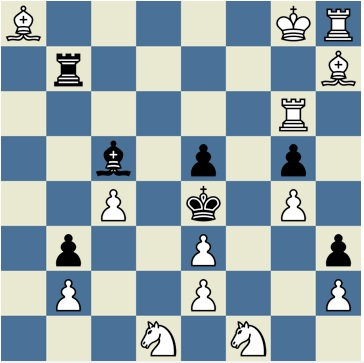# Don't mate in one!What move can white make and not mate in one?

Let $C_1$ be the column you are moving from and $R_1$ be the row you are moving from. And, let $C_2$ be the column you are moving to and $R_2$ be the row you are moving to. (Rows 1-8 and columns 1-8) Finally, concatenate your answer as $C_1$$R_1$$C_2$$R_2$

For example, if you were to take black's pawn with your rook, you'd put your answer as 7675.

×